Courses

# RD Sharma Solutions - Ex-3.3, Decimals, Class 7, Math Class 7 Notes | EduRev

## Class 7: RD Sharma Solutions - Ex-3.3, Decimals, Class 7, Math Class 7 Notes | EduRev

The document RD Sharma Solutions - Ex-3.3, Decimals, Class 7, Math Class 7 Notes | EduRev is a part of the Class 7 Course RD Sharma Solutions for Class 7 Mathematics.
All you need of Class 7 at this link: Class 7

Q1. Divide:

(i) 142.45 by 10
(ii) 54.25 by 10
(iii) 3.45 by 10
(iv) 0.57 by 10
(v) 0.043 by 10
(vi) 0.004 by 10

Solution.

(i) 142.45 by 10

Shifting the decimal point by one place to the left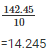(ii) 54.25 by 10

Shifting the decimal point by one place to the left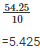(iii) 3.45 by 10

Shifting the decimal point by one place to the left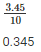(iv) 0.57 by 10

Shifting the decimal point by one place to the left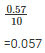(v) 0.043 by 10

Shifting the decimal point by one place to the left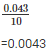(vi) 0.004 by 10

Shifting the decimal point by one place to the left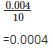Q2. Divide:

(i) 459.5 by 100
(ii) 74.3 by 100
(iii) 5.8 by 100
(iv) 0.7 by 100
(v) 0.48 by 100
(vi) 0.03 by 100

Solution.

(i) 459.5 by 100

Shifting the decimal point by two places to the left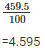(ii) 74.3 by 100

Shifting the decimal point by two places to the left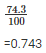(iii) 5.8 by 100

Shifting the decimal point by two places to the left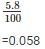(iv) 0.7 by 100

Shifting the decimal point by two places to the left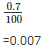(v) 0.48 by 100

Shifting the decimal point by two places to the left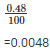(vi) 0.03 by 100

Shifting the decimal point by two places to the left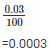Q3. Divide:

(i) 235.41 by 1000
(ii) 29.5 by 1000
(iii) 3.8 by 1000
(iv) 0.7 by 1000

Solution.

(i) 235.41 by 1000

Shifting the decimal point by three places to the left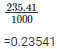(ii) 29.5 by 1000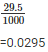(iii) 3.8 by 1000

Shifting the decimal point by three places to the left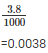(iv) 0.7 by 1000

Shifting the decimal point by three places to the left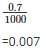Q4. Divide:

(i) 0.45 by 9
(ii) 217.44 by 18
(iii) 319.2 by 2.28
(iv) 40.32 by 9.6
(v) 0.765 by 0.9
(vi) 0.768 by 1.6

Solution.

(i) 0.45 by 9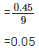(ii) 217.44 by 18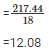(iii) 319.2 by 2.28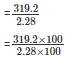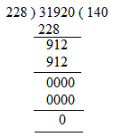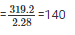(iv) 40.32 by 9.6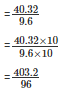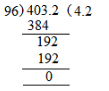= 4.2

(v) 0.765 by 0.9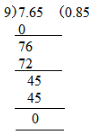= 0.85

(vi) 0.768 by 1.6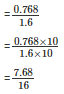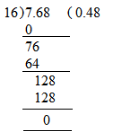= 0.48

Q5. Divide:

(i) 16.64 by 20
(ii) 0.192 by 12

(iii) 163.44 by 24
(iv)  403.2 by 96
(v) 16.344 by 12
(vi) 31.92 by 228

Solution.

(i) 16.64 by 20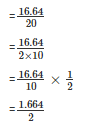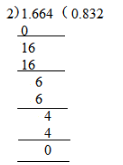= 0.832

(ii) 0.192 by 12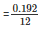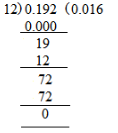= 0.016

(iii) 163.44 by 24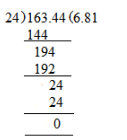= 6.81

(iv)  403.2 by 96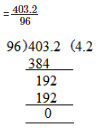= 4.2

(v) 16.344 by 12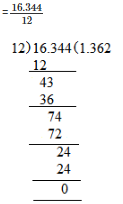= 1.362

(vi) 31.92 by 228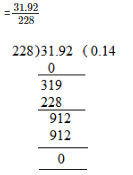= 0.14

Q6. Divide:

(i) 15.68 by 20
(ii) 164.6 by 200
(iii) 403.80 by 30

Solution.

(i) 15.68 by 20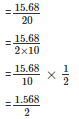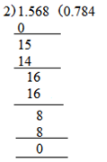= 0.784

(ii) 164.6 by 200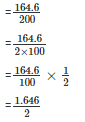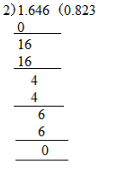= 0.823

(iii) 403.80 by 30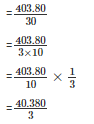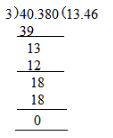= 13.46

Q7. Divide:

(i) 76 by 0.019
(ii) 88 by 0.08
(iii) 148 by 0.074
(iv) 7 by 0.014

Solution.

(i) 76 by 0.019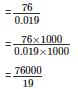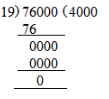=4000

(ii) 88 by 0.08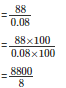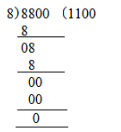=1100

(iii) 148 by 0.074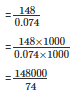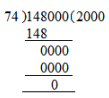= 2000

(iv) 7 by 0.014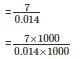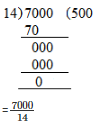Q8. Divide:

(i) 20 by 50
(ii) 8 by 100
(iii) 72 by 576
(iv) 144 by 15

Solution.

(i) 20 by 50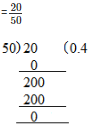(ii) 8 by 100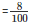By shifting the decimal point to the left=0.08

(iii) 72 by 576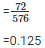(iv) 144 by 15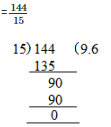= 9.6

Q9. A vehicle covers a distance of 43.2 km in 2.4 litres of petrol. How much distance will it travel in 1 litre of petrol?

Solution.

Distance covered in 2.4 litres of petrol = 43.2km

Distance covered in 1 litre of petrol = 43.2/2.4

= 18km

The distance travelled in 1 litre of petrol is 18 km

Q10. The total weight of some bags of wheat is 1743 kg. If each bag weights 49.8 kg, how many bags are there?

Solution.

Total weight of bags of wheat = 1743kg

Each bag weight = 49.8kg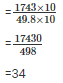Therefore the total numbers of bags are 35

Q11. Shikha cuts 50 m of cloth into pieces of 1.25 m each. How many pieces does she get?

Solution.

Total length of cloth = 50m

Length of each piece of cloth = 1.25m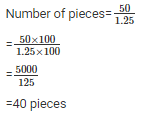Therefore Shikha got 40 pieces

Q12. Each side of a rectangular polygon is 2.5 cm in length. The perimeter of the polygon is 12.5 cm. How many sides does the polygon have?

Solution.

Length of each side of rectangular polygon = 5.2cm

Perimeter of polygon = 12.5cm

No of sides polygon has = 12.5cm

No of sides polygon have = 12.5/2.5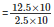= 5

Therefore the sides of the polygon is 5

Q13. The product of two decimals is 42.987. If one of them is 12.46, find the other.

Solution.

We have,

The product of the given decimals = 42.987

one decimal = 12.46

The other decimal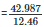= 3.45

The number is 3.45

Q14. The weight of 34 bags of sugar is 3483.3 kg. If all bags weigh equally, find the weight of each bag.

Solution.

Total weight of sugar =  3483.3kg

No of bags = 34

Weight of each bag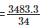= 102.45kg

Therefore weight of each bag is 102.45kg

Q15. How many buckets of equal capacity can be filled from 586.5 litres of water, if each bucket has capacity of 8.5 litres?

Solution.

Capacity of each bucket = 805 litres

Total water available = 586.5litres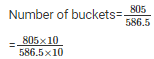= 69

Total number of buckets is 69

The document RD Sharma Solutions - Ex-3.3, Decimals, Class 7, Math Class 7 Notes | EduRev is a part of the Class 7 Course RD Sharma Solutions for Class 7 Mathematics.
All you need of Class 7 at this link: Class 7Use Code STAYHOME200 and get INR 200 additional OFF Use Coupon Code
All Tests, Videos & Notes of Class 7: Class 7

### Top Courses for Class 7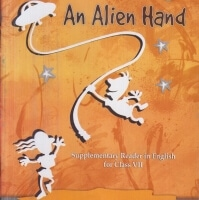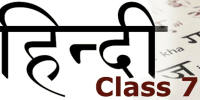## RD Sharma Solutions for Class 7 Mathematics

97 docs

### Top Courses for Class 7Track your progress, build streaks, highlight & save important lessons and more!

,

,

,

,

,

,

,

,

,

,

,

,

,

,

,

,

,

,

,

,

,

,

,

,

,

,

,

,

,

,

;